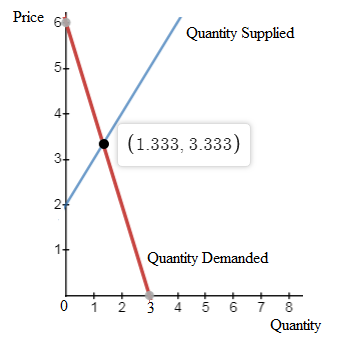# System of Equations:p=6−2qp=2+qPlot both equations on a graph.The values of pp and qq that solve these two equations simultaneously can be seen on the graph asA. the inverse of the slopes of the two linesB. the slopes of the two linesC. the coordinates at which the two lines intersectD. the value of the intercepts of the two lines

Question
22 views
System of Equations:
p=6−2q
p=2+q

Plot both equations on a graph.

The values of pp and qq that solve these two equations simultaneously can be seen on the graph as

A. the inverse of the slopes of the two lines

B. the slopes of the two lines

C. the coordinates at which the two lines intersect

D. the value of the intercepts of the two lines

check_circle

Step 1

The first equation is:

p = 6 - 2q

The second equation is:

p = 2 + q

Plotting both equations on graph:Step 2

The values of price and quantity that solve these two equations simultaneously are the coordinates at which the two lines intersect. This is because at the point of intersection, both...

### Want to see the full answer?

See Solution

#### Want to see this answer and more?

Solutions are written by subject experts who are available 24/7. Questions are typically answered within 1 hour.*

See Solution
*Response times may vary by subject and question.
Tagged in